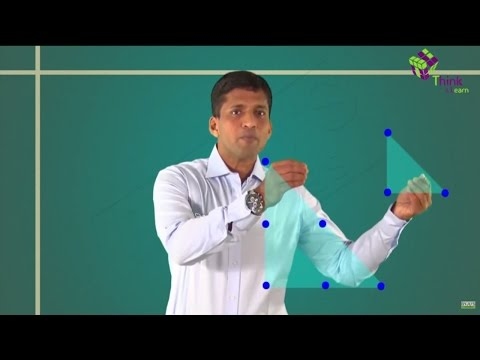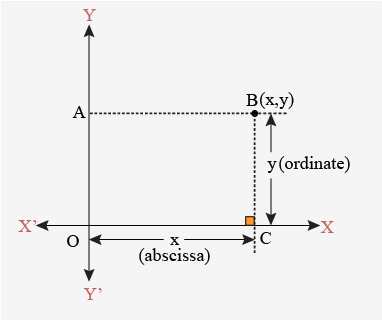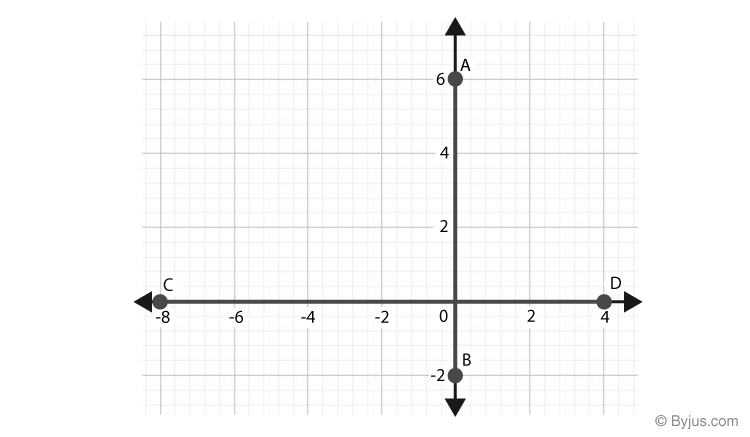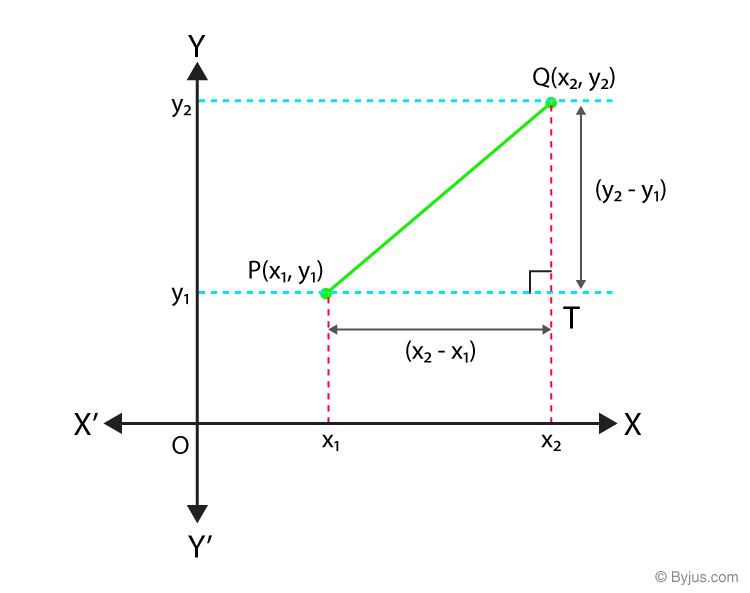# Coordinate Geometry Class 10 Notes

## CBSE Class 10 Maths Coordinate Geometry Notes:-

The complete notes on coordinate geometry class 10 are provided here. Go through the below article and learn the points on the coordinate plane, distance formulas, section formulas and so on with the detailed explanation.

## Basics of Coordinate Geometry

#### For More Information On Basics of Coordinate Geometry, Watch The Below Video.To know more about Coordinate Geometry, visit here.

### Points on a Cartesian Plane

A pair of numbers locate points on a plane called the coordinates.  The distance of a point from the y-axis is known as abscissa or x-coordinate. The distance of a point from the x-axis is called ordinates or y-coordinate.## Distance Formula

### Distance between Two Points on the Same Coordinate Axes

The distance between two points which are on the same axis (x-axis or y-axis), is given by the difference between their ordinates if they are on the y-axis, else by the difference between their abscissa if they are on the x-axis.Distance AB = 6 – (-2) = 8 units

Distance CD = 4 – (-8) = 12 units

### Distance between Two Points Using Pythagoras TheoremLet P(x1y1) and Q(x2y2) be any two points on the cartesian plane.
Draw lines parallel to the axes through P and Q to meet at T.

ΔPTQ  is right-angled at T.

By Pythagoras Theorem,
PQPTQT2

= (x– x1)+ (y– y1)2

PQ = √[x– x1)+ (y– y1)2]

### Distance Formula

Distance between any two points (x1y1) and (x2y2) is given by
= √[x– x1)2+(y– y1)2]
Where d is the distance between the points (x1,y1) and (x2,y2).

To know more about Distance Formula, visit here.

## Section FormulaIf the point P(x, y) divides the line segment joining A(x1y1) and B(x2y2) internally in the ratio m:n, then, the coordinates of P are given by the section formula as:

$$P(x,\ y)=(\frac{mx_{2}+nx_{1}}{m+n},\frac{my_{2}+ny_{1}}{m+n} )$$

To know more about Section Formula, visit here.

### Finding ratio given the points

To find the ratio in which a given point P(x, y) divides the line segment joining A(x1y1) and B(x2y2),

• Assume that the ratio is k : 1
• Substitute the ratio in the section formula for any of the coordinates to get the value of k.
$$x=\frac{kx_{2}+x_{1}}{k+1}$$

When x1x2 and x are known, k can be calculated. The same can be calculated from the y- coordinate also.

### MidPoint

The midpoint of any line segment divides it in the ratio 1 : 1.
The coordinates of the midpoint(P) of line segment joining A(x1y1) and B(x2y2) is given by
$$p(x,\ y)=(\frac{x_{1}+x_{2}}{2},\frac{y_{1}+y_{2}}{2} )$$

### Points of Trisection

To find the points of trisection P and Q which divides the line segment joining
A(x1y1) and B(x2y2) into three equal parts:

i) AP : PB = 1 : 2

$$P=(\frac{x_{2}+2x_{1}}{3},\frac{y_{2}+2y_{1}}{3} )$$

ii) AQ : QB = 2 : 1
$$Q=(\frac{2x_{2}+x_{1}}{3},\frac{2y_{2}+y_{1}}{3} )$$

### Centroid of a triangle

If A(x1y1),B(x2y2) and C(x3y3) are the vertices of a ΔABC, then the coordinates of its centroid(P) is given by
$$p(x,\ y)=(\frac{x_{1}+x_{2}+x_{3}}{3},\frac{y_{1}+y_{2}+y_{3}}{3} )$$

## Area from Coordinates

### Area of a triangle given its vertices

If A(x1y1),B(x2y2) and C(x3y3) are the vertices of a Δ ABC, then its area is given by

A = 1/2[x1(y− y3x2(y− y1x3(y− y2)]
Where A is the area of the Δ ABC.

To know more about Area of a Triangle, visit here.

### Collinearity Condition

If three points A, B and C are collinear and B lies between A and C, then,

• AB + BC = AC.  AB, BC, and AC can be calculated using the distance formula.
• The ratio in which B divides AC, calculated using section formula for both the x and y coordinates separately will be equal.
• Area of a triangle formed by three collinear points is zero.
Test your knowledge on Coordinate Geometry For Class 10

1. NIRMAL JAT

It helped me a lot. thank you

2. Yes it helped me a lot too 😊

3. Divyanshu Maurya

It become much helpful for me.

4. Hari Bhushith

Thank you Byjus! You helped me a ton!

5. Sarah Betty

very helpful thank you !! :))

6. Vijeta S H

Thank you a lot. Helped me a lot. Notes is given so systematically, I actually took Mathematics textbook from my seniors and as the syllabus was reduced even this chapter pages were also removed. So finally I found Byjus notes and this has helped me.
Thank you.